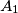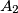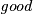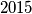IMO Shortlist 2015 problem C3

Kvaliteta:
Avg: 0,0
Težina:
Avg: 7,0

For a finite set$A$ of positive integers, a partition of$A$ into two disjoint nonempty subsets$A_1$ and$A_2$ is$\textit{good}$ if the least common multiple of the elements in$A_1$ is equal to the greatest common divisor of the elements in$A_2$. Determine the minimum value of$n$ such that there exists a set of$n$ positive integers with exactly$2015$ good partitions.

(Ukraine)

Izvor: https://www.imo-official.org/problems/IMO2015SL.pdf